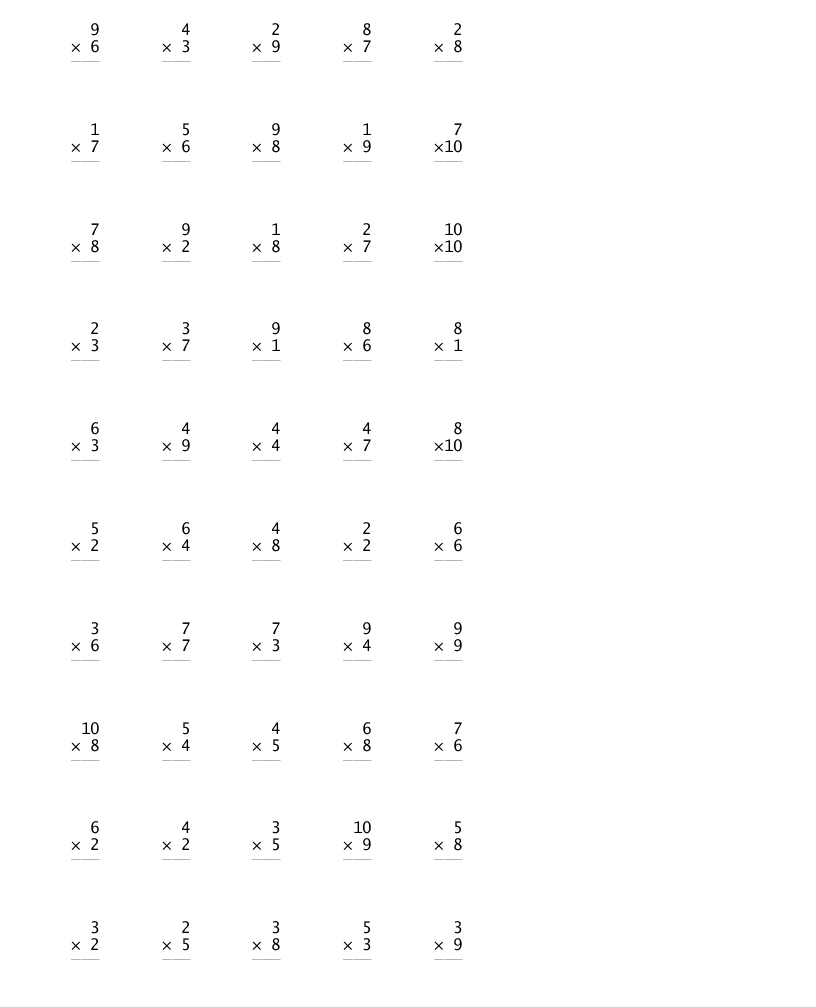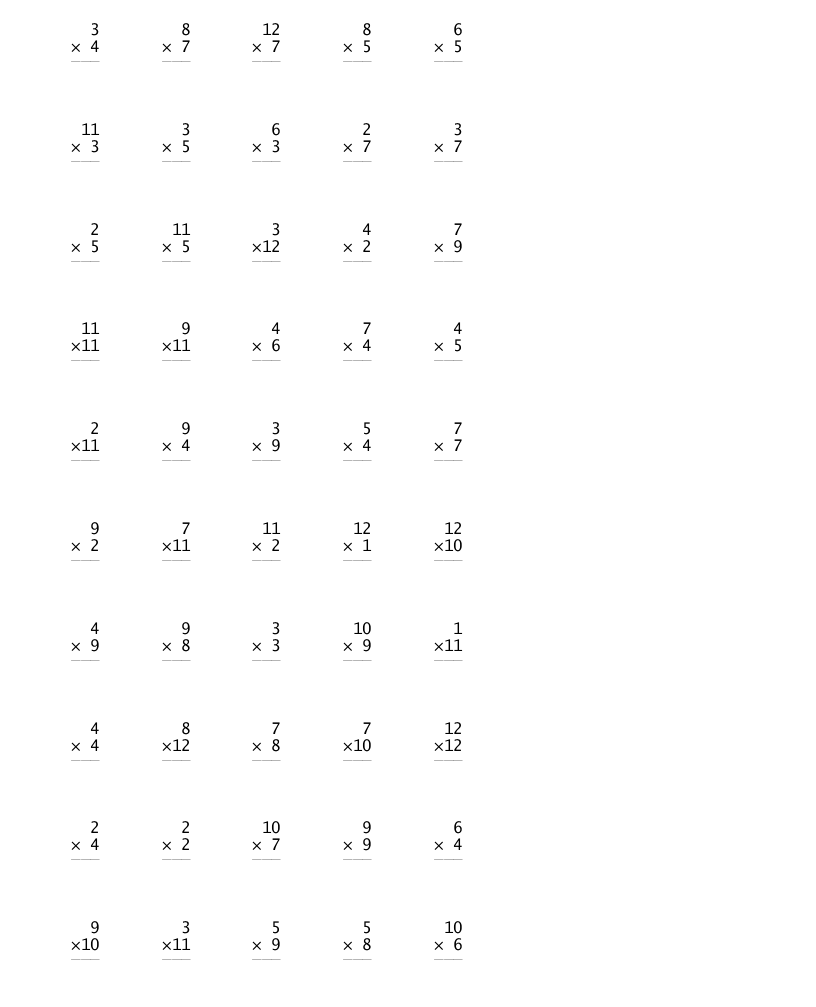The Value of Value Semantics

I am pleased to welcome back Dave Foti to examine some of the ways value semantics work in MATLAB objects and how data copies can be minimized.

As I know some of you may have children who are learning remotely at home now, I thought I would share a MATLAB object I built to help my son practice multiplication. While it is possible to build this into an app, I was looking to make paper tests so this example is designed to make a test that can be printed. Some of you are probably familiar with handle objects in MATLAB or perhaps have used other languages where objects are always references. I want to use my example classes to point out some of the reasons handle or reference semantics are often unnecessary in MATLAB.

Contents

Handles and Values

First, I think of a handle as an object that can access some data that is not part of the object itself but reachable from the object. When I pass around this object, I don’t pass around the actual data, but just an ID that provides the means to reach that data. This means when I change the data owned by the object, I may have passed that ID to many functions. Many variables in various places may now be able to access the same data and see those changes. It often makes sense to represent objects in the real world as handles because you can’t just make copies of real-world objects. For example, if I have a Train object that is designed to track the locations of trains, it might look like this:

classdef Train < handle
properties
Number
Location
end

methods
function p = Train(Number, Location)
p.Number = Number;
p.Location = Location;
end
end
end

No matter how many variables I use to store handles for the same train, I always see the same state.

X = Train(5402, "Boston");
Y = X;  % We can copy the handle or ID, but not the train itself
Y.Location = "New York";
X.Location
ans =
"New York"

What I mean by a value or value semantics, is the notion that when I pass an object to a function the function receives a copy of all the data contained in that object. Similarly, when I assign an object to a new variable, that variable gets a copy of all the data and while two variables may hold identical data, changing data in one variable’s object never changes the data for a different variable. While numbers are the most common forms of values, many kinds of objects can benefit from the same value semantics. Take my arithmetic test for example, it is just a collection of parameters and arithmetic problems.

test = MultTest("NumProblems", 50, "Max", 10)
test =
MultTest with properties:

NumProblems: 50
Max: 10
X: [50×1 double]
Y: [50×1 double]
Z: [50×1 double]
OpSymbol: '×'Now if I want to make a new test that is the same except for going from 1 to 12 instead of 1 to 10, I can assign a new variable to test and then modify it without changing test.

test12 = test;
test12.Max = 12;
test12 = drawProblems(test12);  % recreate and draw the problemsMy original test is not changed:

test
test =
MultTest with properties:

NumProblems: 50
Max: 10
X: [50×1 double]
Y: [50×1 double]
Z: [50×1 double]
OpSymbol: '×'

Semantic Copies are Efficient in MATLAB

Sometimes people worry that making all these copies of objects will lead to using lots of memory. It is important to realize that MATLAB optimizes copies in many ways to make them efficient. When an object is copied from one variable to another, MATLAB simply records this fact, but no data is actually copied. Both variables are actually referencing the same object, but it knows it is linked to multiple variables. This doesn't matter until one of those variables is used to modify the object. Even then, MATLAB makes a shallow copy of the object which means that any matrices inside the object that are not being changed remain shared. When one property is modified, only that property's data is copied, not the data for properties that weren't modified. If we look at the above example again:

test12 = test;
test12.Max = 12;

when test12.Max is changed, no copy of the problems is made because those arrays can still be shared between the objects. When we redraw the problems, only then will new arrays be created for the new problems. With these simple examples, you won't notice the difference, but when objects store very large arrays, efficient copies make a real difference.

Value Semantics Are Clear about Input and Output

One of the features of MATLAB that I've always appreciated is the fact that you can look at a function call and easily tell what the inputs and outputs are, and even if a variable is both an input and an output. Some languages and systems use inputs for outputs and you have to read the function documentation or input argument annotations like "in", "out" and "in/out" to understand how the arguments are used. In MATLAB, inputs are on the right, outputs are on the left and parameters that are in/out appear on both the left and the right. When a variable is used on the left and right side of a function call, MATLAB can often avoid making a copy even if the variable is modified by the function. Let's look at the first few lines of the drawProblems function for ArithmeticTest:

dbtype ArithmeticTest.m 45:50
45            function t = drawProblems(t, withAnswers)
46                arguments
47                    t
48                    withAnswers (1,1) logical = false;
49                end
50                t = genProblems(t);

On line 45, we can see that t is both an input and output. This means that MATLAB can sometimes modify t in place without making a copy but only if the call to the function uses the same variable for the input and output. We can see an example of such a call to a different method on line 50. Even through these multiple levels of function calls, it is possible that t is never actually copied because it is possible that MATLAB can tell that it never needs more than one copy to preserve value semantics.

Below you will find the full program listings for reference:

I'd like to hear about any examples of new value types you've created in MATLAB. Please post any descriptions or links you would like to share in here.

Appendix: Code Files

classdef ArithmeticTest
% ArithmeticTest  Abstract base class for arithmetic tests

properties
% Number of problems in the test
NumProblems (1,1) {mustBeReal, mustBeInteger} = 35;
% Limits the values of operands
Max         (1,1) {mustBeReal, mustBeInteger} = 20;
end
properties (SetAccess = protected)
X
Y
Z
OpSymbol
end

methods(Abstract)
t = genProblems(t)
end

methods
function t = ArithmeticTest(params)
% Assign all the name value pairs to properties of the
% object being constructed.
for s = string(fieldnames(params)')
t.(s) = params.(s);
end
end
end

methods(Access = protected)
function op = getSymbol(t, k)
if isscalar(t.OpSymbol)
op = t.OpSymbol;
else
op = t.OpSymbol(k);
end
end
end

methods
% drawProblems  Draw the problems in a figure that can be printed
% drawProblems(t, true) will print the problems with the answers
% filled in.
arguments
t
end
t = genProblems(t);
if (t.NumProblems <= 48)
jN = 6;
kN = 8;
fontSize = 20;
axPos = [0 0 7 10];
elseif (t.NumProblems <= 80)
jN = 8;
kN = 10;
fontSize = 12;
axPos = [0.55 0 8.5 10.4];
else
jN = 10;
kN = 10;
fontSize = 12;
axPos = [0.55 0 8.5 10.4];
end
f = figure('Units', 'inches', 'Position', [3 0 8.5 11]);
a = axes('Parent', f, 'Units', 'Inches', 'Position', axPos);
a.Visible = 'off';
a.XLim = [0 jN+1];
a.YLim = [0 kN];
n = 1;
for j = 1:jN
for k = 1:kN
if (n > t.NumProblems)
break;
end
x = t.X((j-1)*kN+k);
y = t.Y((j-1)*kN+k);
if (x < 99) && (y < 99)
dx = 2;
dy = 2;
else
dx = 3;
dy = 3;
end
tx1 = text(j-.8, k-.3, ...
sprintf(" %" + dx + "d\n%s%" + dy + "d", ...
x, t.getSymbol(n), y));
tx1.FontName = 'Lucida Console';
tx1.FontSize = fontSize;
tx1.Interpreter = 'none';

if (x < 99) && (y<99)
underlineTxt = '___';
else
underlineTxt = '____';
end
tx2 = text(j-.8, k-.44, underlineTxt);

tx2.FontName = 'Courier New';
tx2.FontSize = fontSize;
tx2.Interpreter = 'none';
tx3 = text(j-.8, k-.7, sprintf(" %"+dx+"d", ...
t.Z((j-1)*jN+k)));
tx3.FontName = 'Courier New';
tx3.FontSize = fontSize;
tx3.Interpreter = 'none';
end
n = n + 1;
end
if (n > t.NumProblems)
break;
end
end
end
end
end

classdef MultTest < ArithmeticTest
% MultTest  Test for multiplication problems

methods
function t = MultTest(params)
arguments
params.?MultTest
end
t@ArithmeticTest(params);
t.OpSymbol = char(215); % Unicode code point 00D7
t = drawProblems(t);
end

function t = genProblems(t)
rng('shuffle');
% numbers from 1 ... t.Max.
A1 = 1:t.Max;
A2 = 1:t.Max;
X = zeros(t.Max^2, 1);
Y = zeros(t.Max^2, 1);
for k = 1:t.Max
for j = 1:t.Max
X((k-1)*t.Max+j,1) = A1(k);
Y((k-1)*t.Max+j,1) = A2(j);
end
end

% Reduce the number of easy questions involving 10 or 1.
idx = find(X == 10);
X(idx(1:5)) = [];
Y(idx(1:5)) = [];
idx = find(Y == 10);
X(idx(1:5)) = [];
Y(idx(1:5)) = [];
idx = find(X == 1);
X(idx(1:5)) = [];
Y(idx(1:5)) = [];
idx = find(Y == 1);
X(idx(1:5)) = [];
Y(idx(1:5)) = [];

% Randomly permute the problems and make sure we have
% enough problems.
numProbs = numel(X);
numSets = ceil(t.NumProblems/numProbs);
t.X = zeros(t.NumProblems, 1);
t.Y = zeros(t.NumProblems, 1);
if numSets > 1
for k = 1:numSets-1
idx = randperm(numProbs, numProbs);
t.X((k-1)*numProbs+1:k*numProbs) = X(idx);
t.Y((k-1)*numProbs+1:k*numProbs) = Y(idx);
end
else
k = 0;
end
idx = randperm(numProbs, numProbs);
t.X(k*numProbs+1:end) = X(idx(1:t.NumProblems-k*numProbs));
t.Y(k*numProbs+1:end) = Y(idx(1:t.NumProblems-k*numProbs));

% Compute the products
t.Z = t.X .* t.Y;
end
end
end

Published with MATLAB® R2020a

|